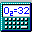##Compute Charge

Location: Program Preferences Window

The program now includes a charge computation algorithm. This has been added predominantly for use in the Formula Finder, but I've included one for use with regular formulas too. The charges for each element can be edited in the Edit elements window while the charges for the abbreviations can be edited in the Edit Abbreviations window.

The method in which charge is computed is different for formulas in the Main Window and the Formula Finder window. This is because formulas in the Formula Finder are simply empirical formulas while those in the Main Window are assumed to be typed as their structure would look. For example, when parentheses are used in the Main Window, a charge correction is employed. Also, hydrogen must be treated specially. In the Main Window the charge of hydrogen is assumed to be +1. This is done so that H2O will have a charge of 2 times 1 minus 2 = 0. However, if the element preceding a hydrogen atom is not a halide (for example, it is carbon, silicon, or any metal), then the charge on hydrogen will be assumed to be -1. Thus, CH3 has an overall charge of 4 minus 3 = 1 and CH4 has a charge of 0.

In the Formula Finder, the charge must be determined simply given an empirical formula. For this reason, a number of assumptions are made. First, all carbon atoms are assumed to be linked by single bonds. If you know you'll have aromatic compounds, then make an abbreviation for C6 and give it a charge of +6. Note that Ph is already the abbreviation for C6H5 (the phenyl group) and it has been given a charge of +1. The second assumption is that Hydrogen is +1. However, if carbon is present, all hydrogens are assumed to be -1. Lastly, if nitrogen is present, the charge is adjusted to assume that each nitrogen takes the place of one hydrogen on a carbon, then any hydrogens that remain must belong to nitrogen, and thus must be positive. With this in mind, the overall charge is further corrected.

Some examples:

 Structural Formula Charge in Main Window Empirical Formula Charge in Formula Finder CH3CH2CH2CH2Cl 0 C4H9Cl 0 ClCH2C(CH3)2CH2 1+ C5H10Cl 1+ CH3CH2CH(NH2)CH2NH2 0 C4H12N2 0 CH3CH2C(ClNH3)CH3 1+ C4H11ClN 1- CH3CH2CH2CH2NH2Cl 1- C4H11ClN 1- CH3CH2COOH 0 C3H6O2 2- CH3CH2CHOO 2- C3H6O2 2- C6H5Cl 8+ C6H5Cl 8+ PhCl 0 PhCl 0

Note: Entering an empirical formula with Nitrogen into the main window will give an erroneous charge since all of the hydrogens will be assumed to belong to carbon and thus be -1 charge. The C4H11ClN empirical formula gives different charges in the Main Window depending on the structural order, while it always gives 1- for the empirical formula. Similarly, C3H6O2 always gives 2- for the overall charge, while the charge can vary in the Main Window. You can enter Ph in the Formula Finder using one of the custom element checkboxes.